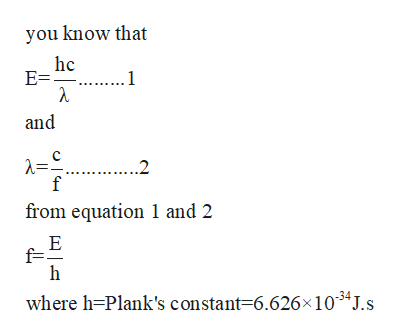# Determine the frequency of photon of light that gives off 2.85 × 10^-19 Joules.

Question
36 views

Determine the frequency of photon of light that gives off 2.85 × 10^-19 Joules.

check_circle

Step 1

Given that,

E = 2.85 ×10-19 J

Now you calculate the frequency of lighthelp_outlineImage Transcriptioncloseyou know that hc E . 1 and 2 f from equation 1 and 2 E f= h where h-Plank's constant=6.626 104J.s fullscreen
Step 2

Now frequency of...

### Want to see the full answer?

See Solution

#### Want to see this answer and more?

Solutions are written by subject experts who are available 24/7. Questions are typically answered within 1 hour.*

See Solution
*Response times may vary by subject and question.
Tagged in

### Atomic structure